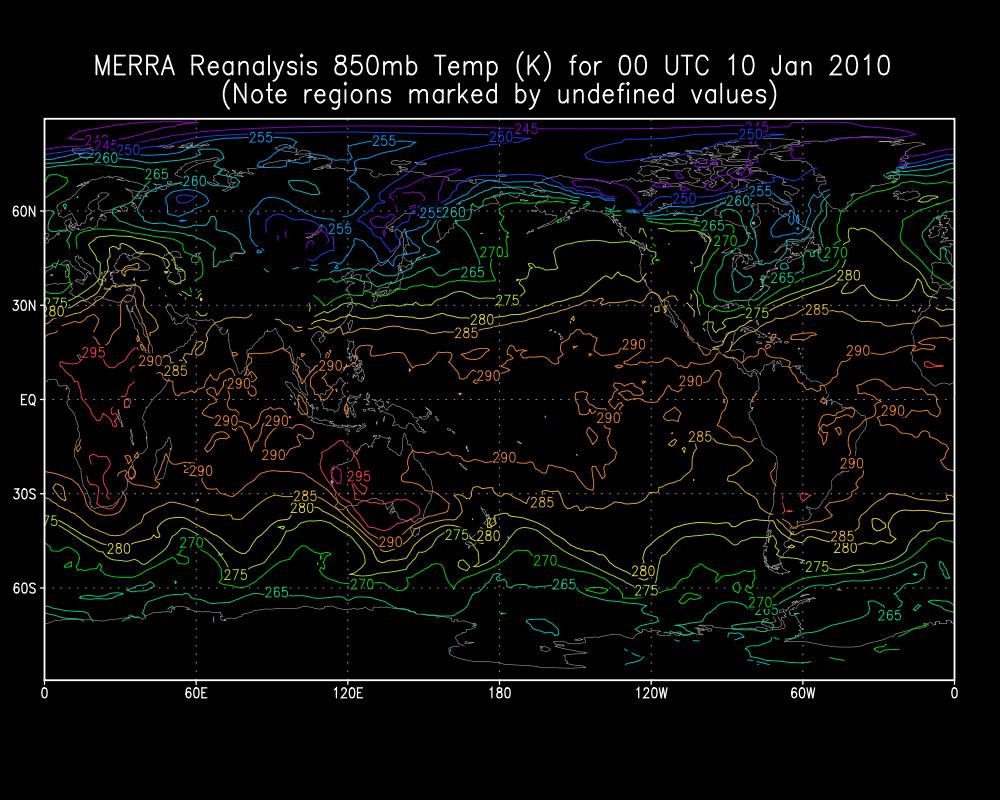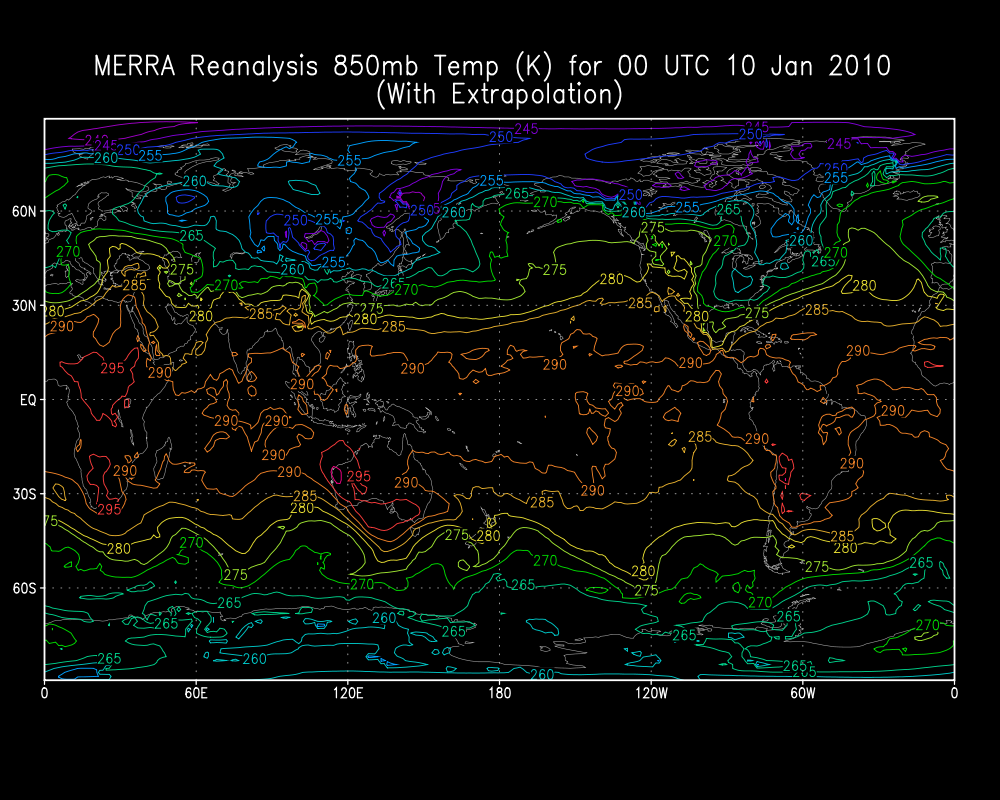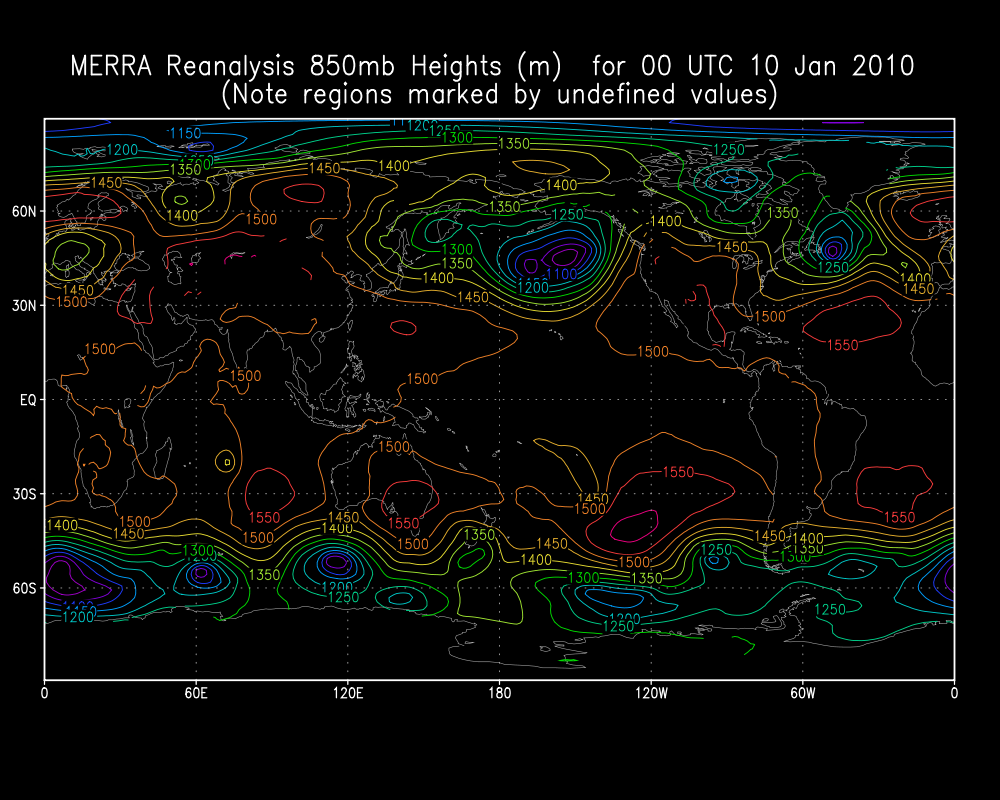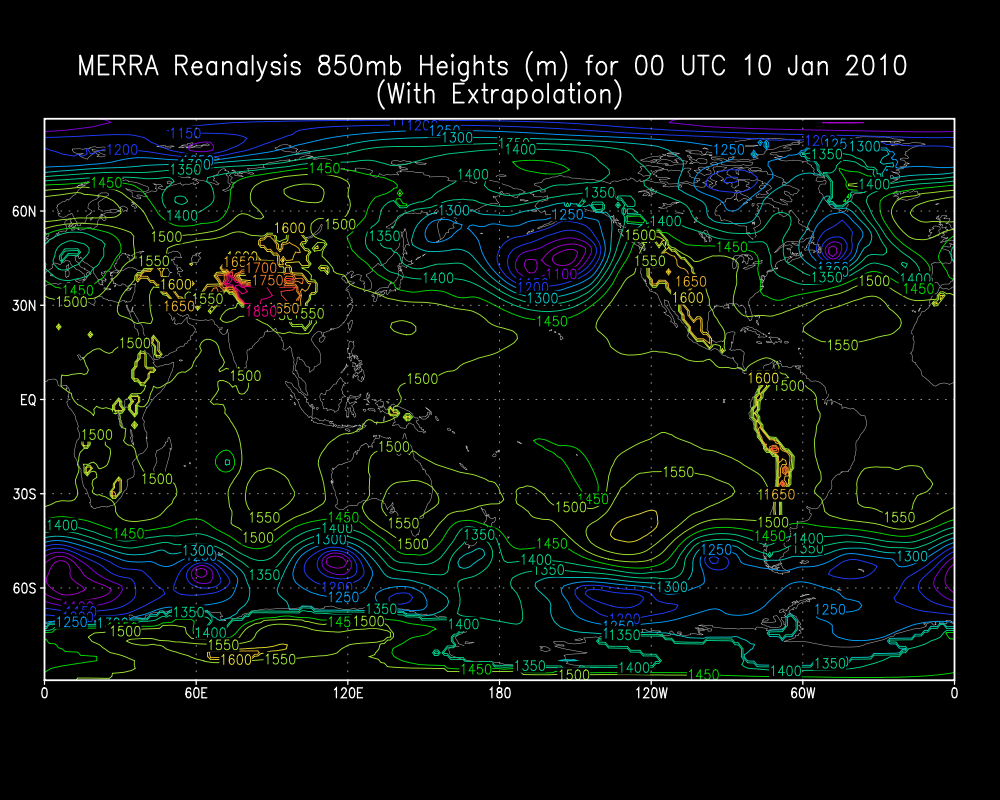# Extrapolation of MERRA Reanalyses to obtain continuous fields

Purpose: To perform basic extrapolations resulting in continuous fields of  NASA MERRA reanalyses for selected model levels where pressure values are greater than the surface pressure (i.e., filling in model grid points "below ground" with defined values). This could be useful where a continuous global field is needed, such as in modeling applications or spherical harmonic analyses. Code samples are included, below.  Geopotential heights are extrapolated by applying the hydrostatic equation and equation of state, as are temperature fields using adiabatic lapse rate approximations (moist or dry).

Background reference from the MERRA FAQs (https://gmao.gsfc.nasa.gov/research/merra/faq.php)

Q. 6 "Why are there such large discrepancies at 1000MB and 850MB between MERRA and other Reanalyses?"

A. "The GEOS5 data assimilation system used to produce MERRA does not (or did not at the time of production) extrapolate data to pressure levels greater than the surface pressure. These grid points are marked by undefined values. The result is that area averages that include these points will not be representative compared to other data sets without additional screening. Time averages, such as monthly means, may also have substantial differences at the edges of topography. The lowest model level data and surface data are available so that users can produce their own extrapolation. A page discussing this issue is available. See http://gmao.gsfc.nasa.gov/research/merra/pressure_surface.php.   "

Below, note regions corresponding to high model topography where contours end due to undefined values in a sample MERRA Reanalysis plot (for example, portions of Rocky Mountains, Andes Mountains, Tibetan Plateau, Antarctica):With extrapolation, the values are "filled in", as this figure shows (below):Here is another example with Geopotential height:And, after extrapolation:Below are sample codes and scripts that perform extrapolation on 5 variables from a MERRA NetCDF file.

Software needed: GrADS, Fortran compiler, Unix/Linux shell environment.

Need a MERRA NetCDF or HDF file along with the following:1.Shell executable extrap.csh:   Runs programs, creates output.  Calls grads scripts and fortran executable***********************************

` `

`#!/bin/csh`

` `

` `

` `

`grads -bpc 'fill_merra_9999.gs'`

` `

`#output tot_merra_filled_9999.prs`

` `

`#view output with check_filled_9999.ctl`

` `

` `

` `

` `

` `

`#Next run Fortran77 executable to do extrapolation:`

` `

` `

` `

`set infile="tot_merra_filled_9999.prs"`

` `

`set outfile="tot_extrapolated.prs"`

` `

`#`

` `

`#Note this code multiplies RH*100`

` `

`extrap_merra << input`

` `

`\$infile`

` `

`\$outfile`

` `

`input`

` `

` `

` `

`#Use this grads control file to check on extrapolated fields:`

` `

`#check_extrapolated.ctl`

` `

` `

` `

`echo All done with extrapolation`

` `*********************************************

2. Two GrADS control files are needed:

check_filled_9999.ctl (views output w/-9999 set as undefined):

DSET ^tot_merra_filled_9999.prs
undef -9999
TITLE MERRA Analyses
XDEF 288 linear -179.375 1.25
YDEF 144 linear -89.375 1.25
ZDEF 17 LEVELS 1000. 925. 850. 700. 600. 500. 400. 300. 250. 200. 150. 100. 70. 50. 30. 20. 10.
TDEF 8 LINEAR 00Z10Jan2010 3hr
VARS 5
h       17  99  geop height (m)
t       17  99  temperature (K)
u       17  99  u-wind (m/s)
v       17  99  v-wind (m/s)
rh      17  99  relative humidity (*100 is %)
ENDVARS

check_extrapolated.ctl (views extrapolated output):

DSET ^tot_extrapolated.prs
undef -9999
TITLE Extrapolated MERRA Analyses
XDEF 288 linear -179.375 1.25
YDEF 144 linear -89.375 1.25
ZDEF 17 LEVELS 1000. 925. 850. 700. 600. 500. 400. 300. 250. 200. 150. 100. 70. 50. 30. 20. 10.
TDEF 8 LINEAR 00Z10Jan2010 3hr
VARS 5
h       17  99  geop height (m)
t       17  99  temperature (K)
u       17  99  u-wind (m/s)
v       17  99  v-wind (m/s)
rh      17  99  relative humidity (%)
ENDVARS

fill_merra_9999.gs is a GrADS script called in extrap.csh.
It specifies "undefined" w/ -9999 using const() and maskout() functions.
A netcdf file in the script was downloaded from the NASA MERRA server
MERRA300.prod.assim.inst3_3d_asm_Cp.20100110.SUB_1.nc
The file contains 17 levels, 3-hourly output, for one day (8 output times):
The file contains the 5 variables that will be saved to a new file with -9999 as the new undefined value.
Variables are geopotential height, temperature, u-wind, v-wind, and RH.
The resulting binary file is tot_merra_filled_9999.prs, viewed with check_filled_9999.ctl

Here is the code for fill_merra_9999.gs:
*Run in extrap.csh using the command:

` `

`'reinit'`

` `

`'sdfopen MERRA300.prod.assim.inst3_3d_asm_Cp.20100110.SUB_1.nc'`` `

` `

`'set x 1 288'`

` ``'set y 1 144'`

` `

`'set gxout fwrite'`

` `

`'set fwrite tot_merra_filled_9999.prs'`

` `

`t=1`

` `

`while (t <= 8)`

` `

` 'set t 't`

` `

`say 'Analysis time is 't`

` `

`*height`

` `

`  z=1`

` `

`  while (z<=17)`

` `

`    'set  z 'z`

` `

`    'd const(maskout(h,h),-9999.,-u)'`

` `

`    z=z+1`

` `

`  endwhile`

` `

`*`

` `

`*Temperature`

` `

`  z=1`

` `

`  while (z<=17)`

` `

`    'set  z 'z`

` `

`    'd const(maskout(t,t),-9999.,-u)'`

` `

`    z=z+1`

` `

`  endwhile`

` `

`*`

` `

`*u-wind`

` `

`  z=1`

` `

`  while (z<=17)`

` `

`    'set  z 'z`

` `

`    'd const(maskout(u,u+1000),-9999.,-u)'`

` `

`    z=z+1`

` `

`  endwhile`

` `

`*`

` `

`*v-wind`

` `

`  z=1`

` `

`  while (z<=17)`

` `

`    'set  z 'z`

` `

`    'd const(maskout(v,v+1000),-9999.,-u)'`

` `

`    z=z+1`

` `

`  endwhile`

` `

`*`

``` *RH   z=1   while (z<=17)     'set  z 'z     'd const(maskout(rh,rh),-9999.,-u)'     z=z+1   endwhile *  t = t + 1 endwhile 'disable fwrite' 'quit' ```

4.  Fortran file extrap_merra.f:

Code to perform extrapolation, called in extrap.csh.

Sample compile (Mac Intel):
ifort -g -mssse3 -assume byterecl -save -extend-source -o extrap_merra extrap_merra.f

Requires input of 5 variables (see above, h,t,u,v,rh)
Replaces -9999. values in MERRA binary (tot_merra_filled_9999.prs) w/ new, extrapolated data
Wind (u,v), Rel Humidity (rh)  use value of grid point lowest to terrain
Temperature (t) extrapolated using adiabatic lapse rate (moist or dry), dT/DP=const
Geop Height (h) extrapolated by hydrostatic eqn and Eqn of State: dZ/DP=-RT/Pg
Resulting binary tot_merra_filled_9999.prs is viewed with check_extrapolated.ctl

` `

`       program extrap_merra`

` `

` `

` `

`c-9-7-12`

` `

`clab purpose is to fill in -9999. values in a MERRA binary w/extrapolated data`

` `

`clab u,v,rh just use value of grid point lowest to terrain`

` `

`clab T extrapolated using adiabatic lapse rate, dT/DP=const, H extrapolated by hydrostatic eqn/eqn of state:`

` `

`clab dZ/DP=-RT/Pg`

` `

`c-9-7-12`

` `

` `

` `

`      parameter(ni=288,nj=144,nk=17,nt=8)  ! array dimensions, nt analysis times`

` `

`      dimension var(ni,nj),u(ni,nj,nk),vgrads(ni,nj)`

` `

`      dimension h(ni,nj,nk),t(ni,nj,nk),v(ni,nj,nk),rh(ni,nj,nk)`

` `

`      dimension pout(nk),tt(ni,nj,nk),rhh(ni,nj,nk)`

` `

`      dimension hh(ni,nj,nk),uu(ni,nj,nk),vv(ni,nj,nk)`

` `

`      character*120 infile,outfile`

` `

` `

` `

` `

` `

`       data pout/10.,20.,30.,50.,70.,100.,150.,200.,`

` `

`     + 250.,300.,400.,500.,600.,700.,850.,925.,1000./   !Reanalysis levels`

` `

` `

` `

` `

` `

` `

` `

`clab read out data for p levels:`

` `

`       do k=1,nk`

` `

`       pout(k)=pout(k)*100.`

` `

`       write(6,*) pout(k)`

` `

`       enddo`

` `

` `

` `

` `

` `

`clab binary file from merra  whose values "below ground" have been`

` `

`clab filled in with -9999. using grads script fill_merra_9999.gs`

` `

` `

` `

`      read(*,'(A120)')infile`

` `

`      open(UNIT=1,FILE=infile,`

` `

`     +access='direct',type='old',form='unformatted',recl=288*144*4)`

` `

` `

``` clab output file:         read(*,'(A120)')outfile       open(UNIT=17,FILE=outfile,      +access='direct',form='unformatted',recl=288*144*4)           irec1=1       irec2=1         do ntime=1,nt       do nvar=1,5       write(6,*)nvar       if(nvar.eq.1) then c Read out all data and flip k-index (to aid in filling in from top-down)           do k=1,nk            read(1,rec=irec1)vgrads !height            do i=1,ni               do j=1,nj                  h(i,j,nk+1-k)=vgrads(i,j)               enddo            enddo            irec1=irec1+1           enddo         elseif(nvar.eq.2)then  !temperature           do k=1,nk            read(1,rec=irec1)vgrads            do i=1,ni               do j=1,nj                  t(i,j,nk+1-k)=vgrads(i,j)               enddo            enddo            irec1=irec1+1           enddo         elseif(nvar.eq.3)then  !u-wind           do k=1,nk            read(1,rec=irec1)vgrads            do i=1,ni               do j=1,nj                  u(i,j,nk+1-k)=vgrads(i,j)               enddo            enddo            irec1=irec1+1           enddo         elseif(nvar.eq.4)then  !v-wind           do k=1,nk            read(1,rec=irec1)vgrads            do i=1,ni               do j=1,nj                  v(i,j,nk+1-k)=vgrads(i,j)               enddo            enddo            irec1=irec1+1           enddo         else                !RH           do k=1,nk            read(1,rec=irec1)vgrads            do i=1,ni               do j=1,nj                  rh(i,j,nk+1-k)=vgrads(i,j)               enddo            enddo ```

```            irec1=irec1+1           enddo       endif       enddo   * c Now extrapolate temperature per lapse rate dt/dp           r=287.0         g=9.81 c       Assume dt/dp=const, where const is such that dt/dz=6.5C/1km (moist adiab approx) c       This is similar to dt/dp=6.5C/100 mb for lowest couple of km           gammadry=9.8/10000.0         gammamoi=6.5/10000.0              do k=1,nk             do i=1,ni               do j=1,nj                  tt(i,j,k)=t(i,j,k)                  if(tt(i,j,k).le.-9999.) tt(i,j,k)=tt(i,j,k-1) + gammamoi*(pout(k)-pout(k-1)) c                 if(tt(i,j,k).le.-9999.) tt(i,j,k)=tt(i,j,k-1) + gammadry*(pout(k)-pout(k-1))               enddo            enddo           enddo   c Now extrapolate to find geopotential height below ground   c       Use dz/dp=-RT/Pg to get geopotential height field z at pressure level p c       working downward from the lowest level above the surface           r=287.0         g=9.81              do k=1,nk             do i=1,ni               do j=1,nj                  hh(i,j,k)=h(i,j,k)                  if(hh(i,j,k).le.-9999.) hh(i,j,k)=hh(i,j,k-1) - ((r*tt(i,j,k))/(pout(k)*g))*(pout(k)-pout(k-1))               enddo            enddo           enddo     c Now extrapolate to find u-wind below ground            do k=1,nk             do i=1,ni               do j=1,nj                  uu(i,j,k)=u(i,j,k)                  if(uu(i,j,k).le.-9999.) uu(i,j,k)=uu(i,j,k-1)               enddo ```

```            enddo           enddo   c Now extrapolate to find v-wind below ground            do k=1,nk             do i=1,ni               do j=1,nj                  vv(i,j,k)=v(i,j,k)                  if(vv(i,j,k).le.-9999.) vv(i,j,k)=vv(i,j,k-1)               enddo            enddo           enddo   c Now extrapolate to find RH below ground            do k=1,nk             do i=1,ni               do j=1,nj                  rhh(i,j,k)=rh(i,j,k)                  if(rhh(i,j,k).le.-9999.) rhh(i,j,k)=rhh(i,j,k-1)               enddo            enddo           enddo     C Now reverse k-index to write out all variables from sfc to top            do k=1,nk  !geopotential height             do i=1,ni               do j=1,nj                  var(i,j)=hh(i,j,nk+1-k)               enddo            enddo            write(17,rec=irec2)var            irec2=irec2+1           enddo                do k=1,nk  !temperature             do i=1,ni               do j=1,nj                  var(i,j)=tt(i,j,nk+1-k)               enddo            enddo            write(17,rec=irec2)var            irec2=irec2+1           enddo              do k=1,nk    !u-wind             do i=1,ni               do j=1,nj                  var(i,j)=uu(i,j,nk+1-k)               enddo ```

```            enddo            write(17,rec=irec2)var            irec2=irec2+1           enddo              do k=1,nk  !v-wind             do i=1,ni               do j=1,nj                  var(i,j)=vv(i,j,nk+1-k)               enddo            enddo            write(17,rec=irec2)var            irec2=irec2+1           enddo              do k=1,nk  ! RH and multiply x100 to get percent             do i=1,ni               do j=1,nj                  var(i,j)=rhh(i,j,nk+1-k)*100.               enddo            enddo            write(17,rec=irec2)var            irec2=irec2+1           enddo   c End iteration of time:        enddo        end ```

Contact: Lee Byerle